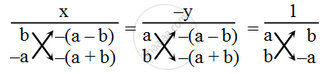# Solve the following system of equations by cross-multiplication method. ax + by = a – b; bx – ay = a + b - Mathematics

Sum

Solve the following system of equations by cross-multiplication method.

ax + by = a – b; bx – ay = a + b

#### Solution

Rewriting the given system of equations, we get

ax + by – (a – b) = 0

bx – ay – (a + b) = 0

By cross-multiplication method, we have\Rightarrow \frac{x}{b\times \{-(a+b)\}-(-a)\times\{-(a-b)\}}=\frac{-y}{-a(a+b)+b(a-b)}=\frac{1}{-a^{2}-{b^{2}

\Rightarrow\frac{x}{-ab-b^{2}-a^{2}+ab}=\frac{-y}{-a^{2}-ab+ab-b^{2}}=\frac{1}{-a^2+b^{2}}

\Rightarrow\frac{x}{-(a^{2}+b^{2})}=\frac{-y}{-(a^{2}+b^{2})}=\frac{1}{-(a^{2}+b^{2})}

\Rightarrow\frac{x}{-(a^{2}+b^{2})}\frac{1}{-(a^{2}+b^{2})}\Rightarrowx=1

and\text{}\frac{-y}{-(a^{2}+b^{2})}\frac{1}{-(a^{2}+b^{2})}\Rightarrow y=-1

Concept: Algebraic Methods of Solving a Pair of Linear Equations - Cross - Multiplication Method
Is there an error in this question or solution?